top of page

### Power Cycle Components/Processes Analysis

This section provides free Power Cycle Components/Processes Analysis.

Power Cycle Components/Processes Analysis covers the following areas:
Compression
Stoichiometric Combustion (Carbon, Hydrogen, Sulfur, Coal, Oil and Gas)
Expansion

Compression

Introduction

This section provides an isentropic compression analysis when the working fluid is air.

Analysis

In the presented compression analysis, only air is considered as the working fluid behaving as a perfect gas -- specific heat has a constant value.  Ideal gas state equation is valid -- pv = RT.

Air enters a compressor at point 1 and it exits the compressor at point 2.  Isentropic compression is considered with no entropy change.

Figure 1 presents a compression schematic layout.Figure 1 - Compression Schematic Layout

Figure 2 presents a compression temperature vs entropy diagram.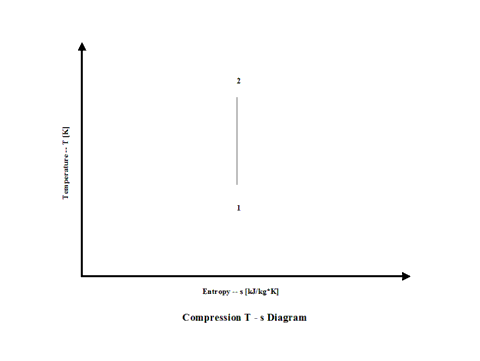Figure 2 - Compression Temperature vs Entropy Diagram

Figure 3 presents compression specific power input requirements for a few typical compression ratio values.  It should be noted that the air enters the compressor at standard ambient conditions of 298 [K] and 1 [atm] of absolute pressure.Figure 3 - Compression Specific Power Input

Figure 4 presents compression power input requirements for two typical compression ratio values and a few different working fluid mass flow rate values for a fixed compression inlet temperature.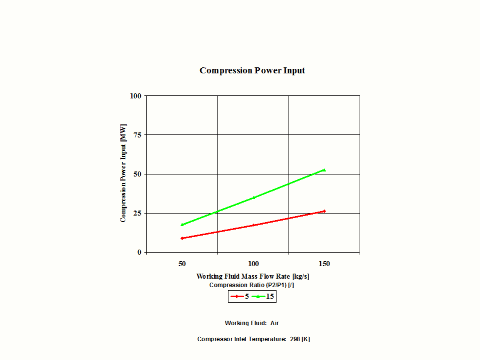Figure 4 - Compression Power Input

One can notice that both compression specific power input and power input increase with an increase in the compression ratio values.  As the working fluid mass flow rate increases for a fixed compression inlet temperature, the compression power input requirements increase too.

Assumptions

Working fluid is air.  There is no friction and heat transfer. Compression is isentropic -- there is no entropy change.  Ideal gas state equation is valid -- pv = RT.  Air behaves as a perfect gas -- specific heat has a constant value.

Governing Equations

T2/T1 = (p2/p1)^(ϰ-1)/ϰ
ϰ = cp/cv
cp - cv = R
pv = RT
w = c
p(T2 - T1)
W = c
p(T2 - T1)m

Input Data

T1 = 298 [K]
p
1 = 1 [atm]
p
2 = 5, 10 and 15 [atm]
R = 0.2867 [kJ/kg*K]
c
p = 1.004 [kJ/kg*K]

ϰ = 1.4 [/]
m = 50, 100 and 150 [kg/s]

Results

Specific Power Input vs Compression Ratio
Compression Inlet Temperature = 298 [K]

Compression
Ratio
[/]

5

10

15

Specific Power
Input
[kW/kg/s]

174

278

349

Power Input vs Compression Ratio for a few Mass Flow Rates
Compression Inlet Temperature = 298 [K]

Power Input
[MW]

Compression Ratio
[/]

5

15

Mass Flow Rate
[kg/s]

50

8.7

17.5

100

17.4

34.9

150

26.0

52.4

Conclusions

Both compression specific power input and power input increase with an increase in the compression ratio values.  As the working fluid mass flow rate increases for a fixed compression inlet temperature, the compression power input requirements increase too.

References

JANAF Thermochemical Data - Tables, 1970

Stoichiometric Combustion (Carbon, Hydrogen, Sulfur, Coal, Oil and Gas)

Introduction

This section provides a combustion analysis for a few typical fuel cases (carbon, hydrogen, sulfur, coal, oil and gas) when the fuel reacts with air at stoichiometric conditions.

Analysis

In the presented combustion analysis, both fuel and air are at standard inlet combustion conditions of 298 [K] and 1 [atm] of absolute pressure. Furthermore, combustion is complete and with no heat loss.

During combustion, a large amount of reactants' chemical energy gets released in the form of thermal energy.

Fuel higher heating value (HHV) or heat of combustion is the difference between the reactants enthalpy value and the combustion products enthalpy value per unit mass amount of fuel at the standard reference temperature, which is 298 [K].

When the reactants specific enthalpy value is equal to the combustion products specific enthalpy value, one can calculate the combustion products flame temperature or adiabatic temperature.

Figure 1 presents how the reactants and combustion products specific enthalpy values change with an increase in the temperature.Figure 1 - Reactants and Combustion Products Specific Enthalpy vs Temperature

Physical properties for both reactants and combustion products are very important and need to be known in order to carry out successful combustion calculations.

Figure 2 presents how the reactants and combustion products species specific enthalpy values change with the temperature.  The physical properties provided in Figure 2 come from the JANAF Thermochemical Data - Tables, 1970.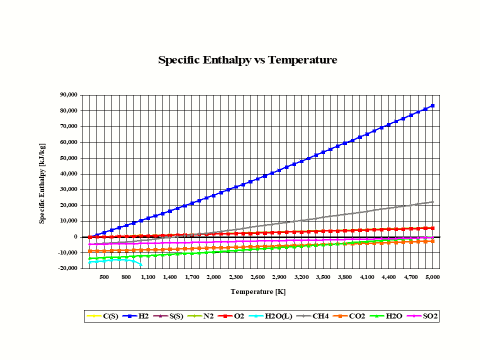Figure 2 - Reactants and Combustion Products Species Specific Enthalpy vs Temperature

It is interesting to note that the specific enthalpy value for basic combustion elements such as carbon (C), hydrogen (H2), sulfur (S), oxygen (O2) and nitrogen (N2) is equal to zero at the standard combustion conditions of 298 [K] and 1 [atm].

Also, it should be mentioned that for ideal gas species, the specific enthalpy values are only dependent on the temperature.
In addition to knowing the reactants and combustion products physical properties, for any kind of combustion analysis and calculations, it is important to know both oxidant and fuel compositions.

Oxidant composition is usually given on the mole/volume basis.  For solid and liquid type fuels, the fuel composition is given on the weight basis for a unit mass amount.  For the gas type fuels, the fuel composition is provided on the mole/volume basis for a unit volume amount.  In this analysis, methane (CH4) is considered as the gas fuel.  In order to keep the combustion analysis simple and straightforward, the CH4 composition is provided on the weight basis.

Table 1 provides the standard air composition.

Table 1 - Oxidant Composition

Oxidant

Air

N

[kg/kg]

0.767

O

[kg/kg]

0.233

N

[kmol/kmol]

0.790

O

[kmol/kmol]

0.210

Table 2 provides the fuel composition.

Table 2 - Fuel Composition

Fuel

Carbon

Hydrogen

Sulfur

Coal

Oil

Gas

C

[kg/kg]

1.000

0.000

0.000

0.780

0.860

-

H

[kg/kg]

0.000

1.000

0.000

0.050

0.140

-

S

[kg/kg]

0.000

0.000

1.000

0.030

0.000

-

N

[kg/kg]

0.000

0.000

0.000

0.040

0.000

-

O

[kg/kg]

0.000

0.000

0.000

0.080

0.000

-

H2O

[kg/kg]

0.000

0.000

0.000

0.020

0.000

-

CH4

[kg/kg]

-

-

-

-

-

1.000

Again, in this combustion analysis, only the stoichiometric combustion is analyzed.  Results of such analysis are provided, including combustion products composition on the weight and mole/volume basis, flame temperature, stoichiometric oxidant to fuel ratio and fuel higher heating value (HHV).

Table 3 provides the combustion products composition on the weight basis.

Table 3 - Combustion Products Composition on the Weight Basis

Fuel

Carbon

Hydrogen

Sulfur

Coal

Oil

Gas

CO2

[kg/kg]

0.295

0.000

0.000

0.249

0.202

0.151

H2O

[kg/kg]

0.000

0.255

0.000

0.041

0.080

0.124

SO2

[kg/kg]

0.000

0.000

0.378

0.005

0.000

0.000

N2

[kg/kg]

0.705

0.745

0.622

0.705

0.718

0.725

O2

[kg/kg]

0.000

0.000

0.000

0.080

0.000

0.000

Table 4 provides the combustion products composition on the volume basis.

Table 4 - Combustion Products Composition on the Volume Basis

Fuel

Carbon

Hydrogen

Sulfur

Coal

Oil

Gas

CO2

[kmol/kmol]

0.210

0.000

0.000

0.170

0.132

0.095

H2O

[kmol/kmol]

0.000

0.347

0.000

0.068

0.129

0.190

SO2

[kmol/kmol]

0.000

0.000

0.210

0.002

0.000

0.000

N2

[kmol/kmol]

0.790

0.653

0.790

0.759

0.739

0.715

O2

[kmol/kmol]

0.000

0.000

0.000

0.080

0.000

0.000

When considering coal, oil and gas as the fuel, coal has the largest amount of CO2 in the combustion products on both weight and mole basis.

Table 5 provides the combustion products flame temperature, stoichiometric oxidant to fuel ratio and fuel higher heating value.

Table 5 - Combustion Products Flame Temperature, Stoichiometric Oxidant to Fuel Ratio
and Fuel Higher Heating Value

Fuel

Carbon

Hydrogen

Sulfur

Coal

Oil

Gas

Flame

Temperature

[K]

2,460

2,525

1,972

2,484

2,484

2,327

Stoichiometric

Oxidant to Fuel

Ratio

[/]

11.444

34.333

4.292

10.487

14.696

17.167

HHV

[Btu/lbm]

14,094

60,997

3,982

14,162

20,660

21,563

Stoichiometric oxidant to fuel ratio is the mass of air required for complete combustion of a unit mass of fuel.  Thus, 1 [kg] of carbon fuel requires 11.444 [kg] of air for complete, ideal combustion.

Today, global warming is becoming more evident and it is being said that it is primarily caused by CO
2 emissions.  A detailed combustion analysis, as it is provided here, can be very useful in determining different fuel and technology scenarios that would result in the reduction of current CO2 emissions.

AssumptionsSpecies Molecular Weight

Species

Molecular Weight

[kg/kmol]

C

12

H2

2

S

32

O2

32

N2

28

CO2

44

H2O

18

S2O

64

CH4

16

Stoichiometric Oxidant and Combustion Products

Mole Flow Rates for 1 [kmol] of Fuel Species

Fuel

Carbon

Hydrogen

Sulfur

Coal

Oil

Gas

Fuel

Composition

Oxidant

Composition

C

[kmol]

1

0

0

1

1

0

H2

[kmol]

0

1

0

1

1

0

S

[kmol]

0

0

1

1

0

0

CH4

[kmol]

0

0

0

0

0

1

O2

[kmol]

1

0.5

1

2.5

1.5

2

N2

[kmol]

3.762

1.881

3.762

9.404

5.642

7.524

CO2

[kmol]

1

0

0

1

1

1

Combustion Products

Composition

H2O

[kmol]

0

1

0

1

1

2

SO2

[kmol]

0

0

1

1

0

0

O2

[kmol]

0

0

0

0

0

0

N2

[kmol]

3.762

1.881

3.762

9.404

5.642

7.524

Stoichiometric Oxidant to Fuel Ratio, Oxidant and Combustion Products

Mass Flow Rates for 1 [kmol] of Fuel Species

Fuel

Carbon

Hydrogen

Sulfur

Coal

Oil

Gas

Fuel

Composition

Oxidant

Composition

C

[kg]

12

0

0

12

12

0

H2

[kg]

0

2

0

2

2

0

S

[kg]

0

0

32

32

0

0

CH4

[kg]

0

0

0

0

0

16

O2

[kg]

32

16

32

80

48

64

N2

[kg]

105.336

52.668

105.336

263.312

157.976

210.672

CO2

[kg]

44

0

0

44

44

44

Combustion Products

Composition

H2O

[kg]

0

18

0

18

18

36

SO2

[kg]

0

0

64

64

0

0

O2

[kg]

0

0

0

0

0

0

N2

[kg]

105.336

52.668

105.336

263.312

157.976

210.672

Stoichimetric

Oxidant to Fuel

Ratio

[/]

11.444

34.333

4.292

7.463

14.712

17.167

Both fuel and air are at standard inlet combustion conditions of 298 [K] and 1 [atm] of absolute pressure.

Furthermore, combustion is complete and with no heat loss.

Governing Equations

Fuel higher heating value (HHV) or heat of combustion is the difference between the reactants enthalpy value and the combustion products enthalpy value per unit mass amount of fuel at the standard reference temperature, which is 298 [K].

When the reactants specific enthalpy value is equal to the combustion products specific enthalpy value, one can calculate the combustion products flame temperature or adiabatic temperature.

Input Data

Table 1 - Oxidant Composition

Oxidant

Air

N

[kg/kg]

0.767

O

[kg/kg]

0.233

N

[kmol/kmol]

0.790

O

[kmol/kmol]

0.210

Table 2 - Fuel Composition

Fuel

Carbon

Hydrogen

Sulfur

Coal

Oil

Gas

C

[kg/kg]

1.000

0.000

0.000

0.780

0.860

-

H

[kg/kg]

0.000

1.000

0.000

0.050

0.140

-

S

[kg/kg]

0.000

0.000

1.000

0.030

0.000

-

N

[kg/kg]

0.000

0.000

0.000

0.040

0.000

-

O

[kg/kg]

0.000

0.000

0.000

0.080

0.000

-

H2O

[kg/kg]

0.000

0.000

0.000

0.020

0.000

-

CH4

[kg/kg]

-

-

-

-

-

1.000

Results

Table 3 - Combustion Products Composition on the Weight Basis

Fuel

Carbon

Hydrogen

Sulfur

Coal

Oil

Gas

CO2

[kg/kg]

0.295

0.000

0.000

0.249

0.202

0.151

H2O

[kg/kg]

0.000

0.255

0.000

0.041

0.080

0.124

SO2

[kg/kg]

0.000

0.000

0.378

0.005

0.000

0.000

N2

[kg/kg]

0.705

0.745

0.622

0.705

0.718

0.725

O2

[kg/kg]

0.000

0.000

0.000

0.080

0.000

0.000

Table 4 - Combustion Products Composition on the Volume Basis

Fuel

Carbon

Hydrogen

Sulfur

Coal

Oil

Gas

CO2

[kmol/kmol]

0.210

0.000

0.000

0.170

0.132

0.095

H2O

[kmol/kmol]

0.000

0.347

0.000

0.068

0.129

0.190

SO2

[kmol/kmol]

0.000

0.000

0.210

0.002

0.000

0.000

N2

[kmol/kmol]

0.790

0.653

0.790

0.759

0.739

0.715

O2

[kmol/kmol]

0.000

0.000

0.000

0.080

0.000

0.000

Table 5 - Combustion Products Flame Temperature, Stoichiometric Oxidant to Fuel Ratio
and Fuel Higher Heating Value

Fuel

Carbon

Hydrogen

Sulfur

Coal

Oil

Gas

Flame

Temperature

[K]

2,460

2,525

1,972

2,484

2,484

2,327

Stoichiometric

Oxidant to Fuel

Ratio

[/]

11.444

34.333

4.292

10.487

14.649

17.167

HHV

[Btu/lbm]

14,094

60,997

3,982

14,162

20,660

21,563

Figures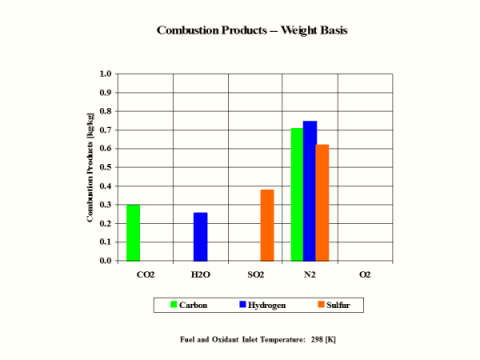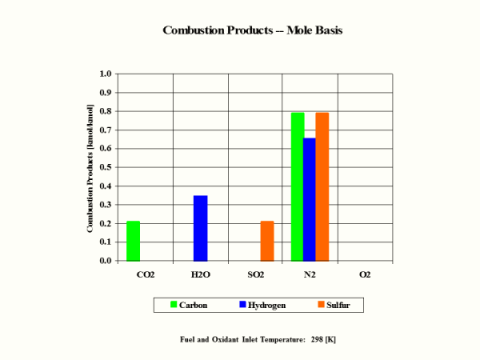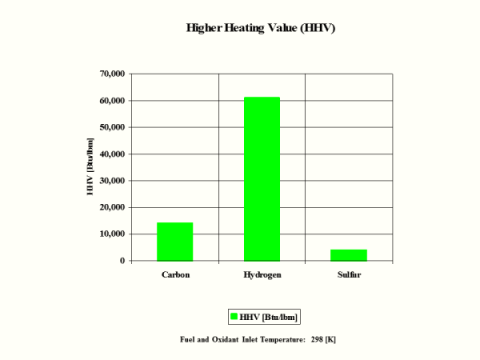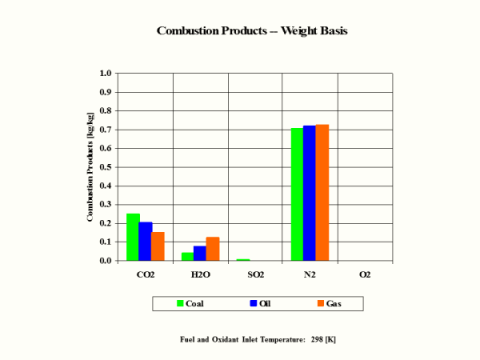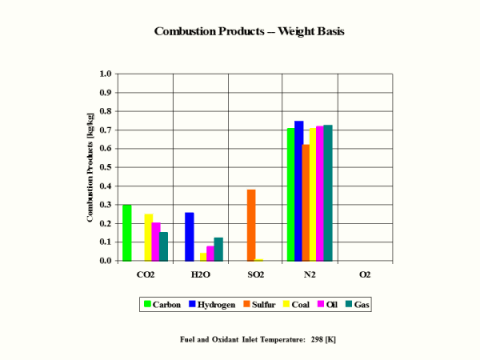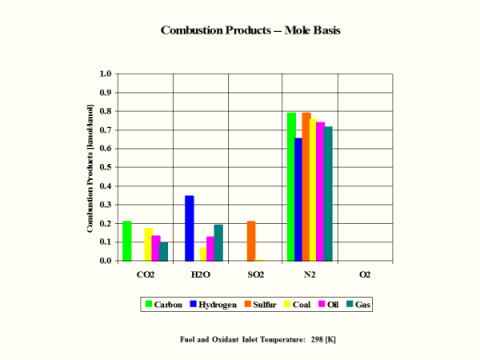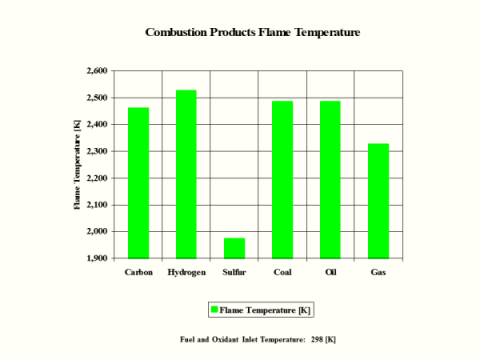Conclusions

Hydrogen as the fuel has the highest flame temperature, requires the most mass amount of oxidant/air in order to have complete combustion per unit mass amount of fuel and the largest fuel higher heating value.

When hydrogen reacts with oxidant/air, there is no CO2 present in the combustion products.

References

JANAF Thermochemical Data - Tables, 1970

Expansion

Introduction

This section provides an isentropic expansion analysis when the working fluid is air.

Analysis

In the presented expansion analysis, only air is considered as the working fluid behaving as a perfect gas -- specific heat has a constant value.  Ideal gas state equation is valid -- pv = RT.

Air enters a turbine at point 1 and it exits the turbine at point 2.  Isentropic expansion is considered with no entropy change.

Figure 1 presents an expansion schematic layout.Figure 1 - Expansion Schematic Layout

Figure 2 presents an expansion temperature vs entropy diagram.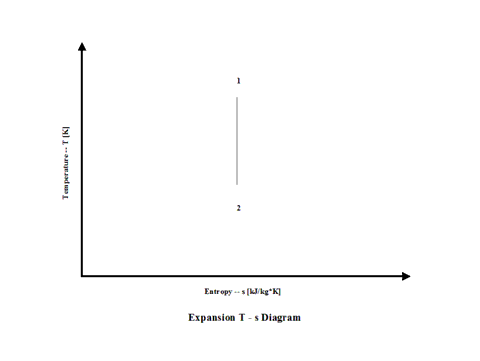Figure 2 - Expansion Temperature vs Entropy Diagram

Figure 3 presents expansion specific power output values for a few typical expansion ratio values.  It should be noted that the air enters the turbine at the temperature of 1,500 [K] and the turbine exhaust pressure is always equal to the standard ambient pressure -- 1 [atm] of absolute pressure.Figure 3 - Expansion Specific Power Output

Figure 4 presents expansion power output values for two typical expansion ratio values and a few different working fluid mass flow rate values for a fixed expansion inlet temperature.Figure 4 - Expansion Power Output

One can notice that both expansion specific power output and power output increase with an increase in the expansion ratio values.  As the working fluid mass flow rate increases for a fixed expansion inlet temperature, the expansion power output values increase too.

Assumptions

Working fluid is air.  There is no friction and heat transfer. Expansion is isentropic -- there is no entropy change.  Ideal gas state equation is valid -- pv = RT. Air behaves as a perfect gas -- specific heat has a constant value.

Governing Equations

T1/T2 = (p1/p2)^(ϰ-1)/ϰ
ϰ = cp/cv
cp - cv = R
pv = RT
w = c
p(T1 - T2)
W = c
p(T1 - T2)m

Input Data

T1 = 1,500 [K]
p
1 = 5, 10 and 15 [atm]
p
2 = 1 [atm]
R = 0.2867 [kJ/kg*K]
c
p = 1.004 [kJ/kg*K]

ϰ = 1.4 [/]
m = 50, 100 and 150 [kg/s]

Results

Specific Power Output vs Expansion Ratio
Expansion Inlet Temperature = 1,500 [K]

Compression
Ratio
[/]

5

10

15

Specific Power
Input
[kW/kg/s]

174

278

349

Power Output vs Expansion Ratio for a few Mass Flow Rates
Expansion Inlet Temperature = 1,500 [K]

Power Output
[MW]

Compression Ratio
[/]

5

15

Mass Flow Rate
[kg/s]

50

27.8

40.6

100

55.5

81.1

150

83.3

121.7

Conclusions

Both expansion specific power output and power output increase with an increase in the expansion ratio values.  As the working fluid mass flow rate increases for a fixed expansion inlet temperature, the expansion power output values increase too.

References

JANAF Thermochemical Data - Tables, 1970

bottom of page